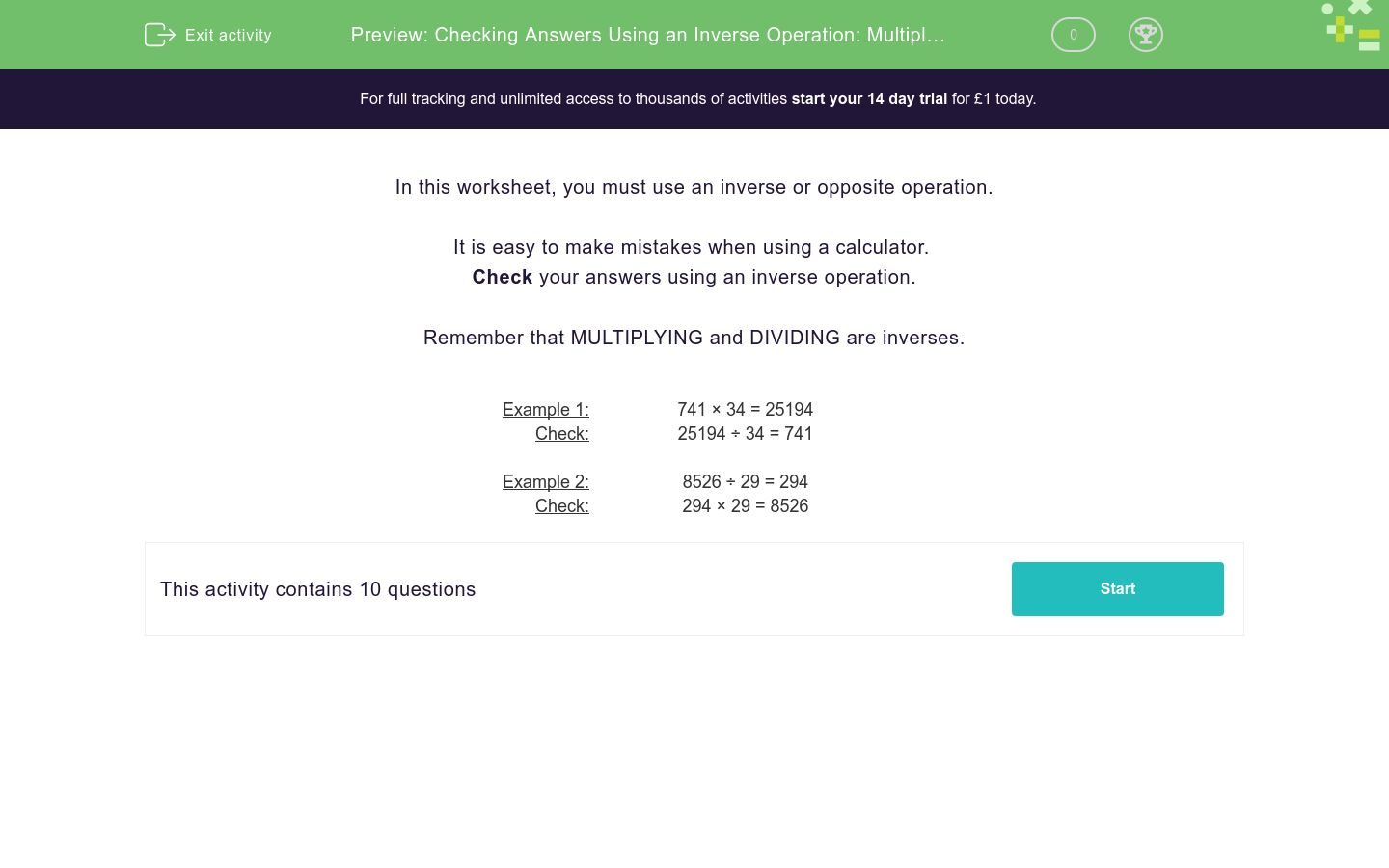# Checking Answers Using an Inverse Operation: Multiplying or Dividing

In this worksheet, students check answers by carrying out an inverse operation.Key stage:  KS 3

Curriculum topic:   Number

Curriculum subtopic:   Use Relationships Between Operations

Difficulty level:### QUESTION 1 of 10

In this worksheet, you must use an inverse or opposite operation.

It is easy to make mistakes when using a calculator.

Remember that MULTIPLYING and DIVIDING are inverses.

 Example 1: 741 × 34 = 25194 Check: 25194 ÷ 34 = 741 Example 2: 8526 ÷ 29 = 294 Check: 294 × 29 = 8526

Check the answer using an inverse operation for the given operation.

3976 ÷ 28 = 142
142 × 28 =

Check the answer using an inverse operation for the given operation.

307 × 596 = 182972
182972 ÷ 596 =

Check the answer using an inverse operation for the given operation.

11592 ÷ 56 = 207
207 × 56 =

Check the answer using an inverse operation for the given operation.

993 × 450 = 446850
446850 ÷ 450 =

Check the answer using an inverse operation for the given operation.

22015 ÷ 85 = 259
259 × 85 =

Check the answer using an inverse operation for the given operation.

624 × 844 = 526656
526656 ÷ 844 =

Check the answer using an inverse operation for the given operation.

26754 ÷ 78 = 343
343 × 78 =

Check the answer using an inverse operation for the given operation.

777 × 821 = 637917
637917 ÷ 821 =

Check the answer using an inverse operation for the given operation.

14880 ÷ 96 = 155
155 × 96 =

Check the answer using an inverse operation for the given operation.

3950 ÷ 25 = 158
158 × 25 =
• Question 1

Check the answer using an inverse operation for the given operation.

3976 ÷ 28 = 142
142 × 28 =
3976
• Question 2

Check the answer using an inverse operation for the given operation.

307 × 596 = 182972
182972 ÷ 596 =
307
• Question 3

Check the answer using an inverse operation for the given operation.

11592 ÷ 56 = 207
207 × 56 =
11592
• Question 4

Check the answer using an inverse operation for the given operation.

993 × 450 = 446850
446850 ÷ 450 =
993
• Question 5

Check the answer using an inverse operation for the given operation.

22015 ÷ 85 = 259
259 × 85 =
22015
• Question 6

Check the answer using an inverse operation for the given operation.

624 × 844 = 526656
526656 ÷ 844 =
624
• Question 7

Check the answer using an inverse operation for the given operation.

26754 ÷ 78 = 343
343 × 78 =
26754
• Question 8

Check the answer using an inverse operation for the given operation.

777 × 821 = 637917
637917 ÷ 821 =
777
• Question 9

Check the answer using an inverse operation for the given operation.

14880 ÷ 96 = 155
155 × 96 =
14880
• Question 10

Check the answer using an inverse operation for the given operation.

3950 ÷ 25 = 158
158 × 25 =
3950
---- OR ----

Sign up for a £1 trial so you can track and measure your child's progress on this activity.

### What is EdPlace?

We're your National Curriculum aligned online education content provider helping each child succeed in English, maths and science from year 1 to GCSE. With an EdPlace account you’ll be able to track and measure progress, helping each child achieve their best. We build confidence and attainment by personalising each child’s learning at a level that suits them.

Get started